Directions for questions 1 to 4 : Study the following graph and answer the question that follow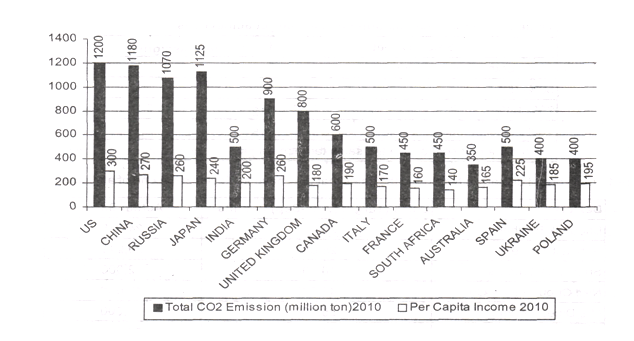Question 1.  If the world energy council formulates a norm for high emission countries to reduce their emission each year by 12.5% for the next two years then what would be the ratio of CO2 emission to per capita income of US, China and Japan after two years. The per capita income of China, Japan and US is expected to increase every year by 4%, 3% and 2% respectively.
A. 3.5, 3.8, 4.1
B. 3.4, 3.5, 3.
C. 2.9, 3.1, 3.4
D. None

Question 2.  If US and China decide to buy carbon credits, from Spain and Ukraine to make up for their high emissions, then in how many years US and China would be able to bring down its ratio of CO2emission (million ton) to per capita income to world standard benchmark of 0.75. (per capita income of the given countries remain same, 0.5 CO2 emissions,[million ton] is compensated by purchase of 1.25 units of carbon credit, and a country can buy carbon credit units in three lots of 15, 20 and 30 units in a single year.)
A. 3.8 years
B. 38 years
C. 30 years
D. None

Question 3.  France, South Africa, Australia, Ukraine and Poland form an energy consortium which declares CO2 emission of 350 million ton per annum as standard benchmark. The energy consortium decides to sell their carbon emission savings against the standard benchmark to high carbon emission countries. It is expected that the per capita income of each country of the energy consortium increases by 2%, 2.5% and 3.5% p.a. for the next three years respectively. The ratio of CO2 emission to per capita income of each energy consortium country reduces by 50% and remains constant for the next three years. By selling 0.5 CO2emissions [million ton] the energy consortium earns 1.25 carbon credits, then determine the total credits earned by energy consortium in three years.
A. 3560
B.4506
C. 5060
D. None

Coemission to per capita income ratio for US after two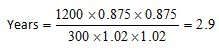CO2 emission to per capita income ratio for China after two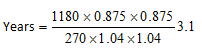CO0 emission to per capita income ratio for Japan after two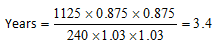Standard benchmark ratio = 0.75

So CO2 emission for USA must be 225

Hence, it has to buy 975 × 2.5 = 2437.5 carbon credit units.

Similarly CO2 emission for China must be 202.5

Hence, It has to buy 977.5 × 2.5 = 2443.75 carbon credit units

In one year a country can buy 15 + 20 + 30 = 65 carbon credit units

Time taken by USA to achieve standard benchmark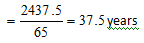Time taken by China to achieve standard benchmark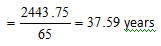Hence, required number of years is 38.

Time combined per capita income of all five countries in 2010 is 845.

So, per capita income in 2011 = 861.9

2011 = 883.44

2013 = 914.37

The ratio of CO2 emission to per capita income e in 2010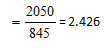The ratio for next three years = 0.5 × 2.426 = 1.213

⇒ CO2emission of 2011 = 861.9 × 1.213 = 1045.48

⇒ CO2 emission of 2012 = 883.44 × 1.213 = 1071.61

⇒ CO2 emission of 2013 = 914.37 × 1.213 = 1109.13

The benchmark emission of CO2 = 350 × 5 = 1750 million tonne

Savings for 2011 = 704.52

Savings for 2012 = 678.39

Savings for 2013 = 640.87

Hence, total carbon credits earned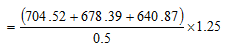= 5059.45 = 5060 approximately.